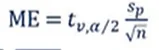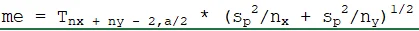16 Nov 2022

Posted on:

14 Nov 2022

0

# 8:21 ME equation discrepancy

the first below equation is shown on the screen but i have written down the second equation below. What is the reason for this discrepancy? it appears the second equation was what was used in the excel sheet. I'm already a bit slow with this class to begin with so any help would be appreciated.Instructor
Posted on:

16 Nov 2022

0

Hi Nicholas,
thanks for reaching out! I believe the difference in the formula's comes mainly from the fact that the second one is for two samples - x and y. The first assumes one sample population. The other difference is in notation, in the first example you have the sigma divided by the square root of the population size, in the second you have the sigma divided by the population size(for both populations) to the power of 1/2, which basically shows the same thing. In statistics you'll often encounter different notations. Another example would be to see large N, instead of n, again showing the same entity which is the population size. I agree that it seems very confusing, but with more practice, you'll get used to the different notations and get the hang of the one vs two samples scenarios.
In the meantime, if you have any other questions, let us know in the Q & A section.

Happy learning,
365 Eli